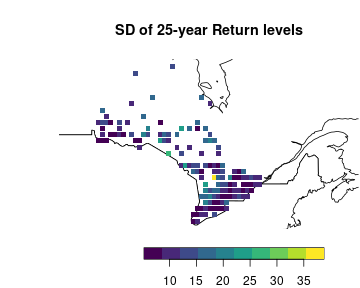# A Guide to the SpatialGEV Package

## Introduction to the GEV-GP Model

The generalized extreme value (GEV) distribution is often used to analyze sequences of maxima within non-overlapping time periods. An example of this type of data is the monthly maximum rainfall levels recorded over years at a weather station. Since there are typically a large number of weather stations within a country or a state, it is more ideal to have a model that can borrow information from nearby weather stations to increase inference and prediction accuracy. Such spatial information is often pooled using the Gaussian process.

The GEV-GP model is a hierarchical model with a data layer and a spatial random effects layer. Let $${\boldsymbol{x}}_1, \ldots, {\boldsymbol{x}}_n \in \mathbb{R}^2$$ denote the geographical coordinates of $$n$$ locations, and let $$y_{ik}$$ denote the extreme value measurement $$k$$ at location $$i$$, for $$k = 1, \ldots, n_i$$. The data layer specifies that each observation $$y_{ik}$$ has a generalized extreme value distribution, denoted by $$y \sim \mathrm{GEV}(a, b, s)$$, whose CDF is given by $\begin{equation} F(y\mid a, b, s) = \begin{cases} \exp\left\{-\left(1+s\frac{y-a}{b}\right)^{-\frac{1}{s}}\right\} \ \ &s\neq 0,\\ \exp\left\{-\exp\left(-\frac{y-a}{b}\right)\right\} \ \ &s=0, \end{cases} \label{eqn:gev-distn} \end{equation}$ where $$a\in\mathbb{R}$$, $$b>0$$, and $$s\in\mathbb{R}$$ are location, scale, and shape parameters, respectively. The support of the GEV distribution depends on the parameter values: $$y$$ is bounded below by $$a-b/s$$ when $$s>0$$, bounded above by $$a-b/s$$ when $$s<0$$, and unbounded when $$s=0$$. To capture the spatial dependence in the data, we assume some or all of the GEV parameters in the data layer are spatially varying. Thus they are introduced as random effects in the model.

A Gaussian process $$z({\boldsymbol{x}})\sim \mathcal{GP}(\mu({\boldsymbol{x}}_{cov}), k({\boldsymbol{x}}, {\boldsymbol{x}}'))$$ is fully characterized by its mean $$\mu({\boldsymbol{x}}_{cov})$$ and kernel function $$k({\boldsymbol{x}}, {\boldsymbol{x}}') = {\mathrm{Cov}}( z({\boldsymbol{x}}), z({\boldsymbol{x}}') )$$, which captures the strength of the spatial correlation between locations. The mean is a function of parameters $$\boldsymbol{\beta}$$ and covariates $${\boldsymbol{x}}_{cov}=(x_1,\ldots,x_p)'$$. We assume that given the locations, the data follow independent GEV distributions each with their own parameters. The complete GEV-GP hierarchical model then becomes \begin{equation} \begin{aligned} y_{ik} \mid a({\boldsymbol{x}}_i), b({\boldsymbol{x}}_i), s & {\overset{ind}{\sim}}\mathrm{GEV}\big( a({\boldsymbol{x}}_i), \exp( b({\boldsymbol{x}}_i) ), \exp(s)\big)\\ a({\boldsymbol{x}}) \mid \boldsymbol{\beta}_a, {\boldsymbol{\theta}}_a &\sim \mathcal{GP}\big( {\boldsymbol{X}}_a\boldsymbol{\beta}_a, k({\boldsymbol{x}}, {\boldsymbol{x}}' \mid {\boldsymbol{\theta}}_a) \big)\\ log(b)({\boldsymbol{x}}) \mid \boldsymbol{\beta}_b, {\boldsymbol{\theta}}_b &\sim \mathcal{GP}\big( {\boldsymbol{X}}_b\boldsymbol{\beta}_b, k({\boldsymbol{x}}, {\boldsymbol{x}}' \mid {\boldsymbol{\theta}}_b) \big)\\ s({\boldsymbol{x}}) \mid \boldsymbol{\beta}_s, {\boldsymbol{\theta}}_s &\sim \mathcal{GP}\big( {\boldsymbol{X}}_s\boldsymbol{\beta}_s, k({\boldsymbol{x}}, {\boldsymbol{x}}' \mid {\boldsymbol{\theta}}_a) \big). \end{aligned} \end{equation} In this package, a uniform prior $$\pi({\boldsymbol{\theta}}) \propto 1$$ is specified on the fixed effect and hyperparameters ${\boldsymbol{\theta}}=(s, \boldsymbol{\beta}_a, \boldsymbol{\beta}_b, \boldsymbol{\beta}_s, {\boldsymbol{\theta}}_a, {\boldsymbol{\theta}}_b, {\boldsymbol{\theta}}_s).$

## What Does SpatialGEV Do?

The package provides an interface to estimate the approximate joint posterior distribution of the spatial random effects $$a$$ and $$b$$ in the GEV-GP model. The main functionalities of the package are:

• Method to fit the GEV-GP model and sample from the approximate joint posterior distribution of $$a$$ and $$b$$

• Method to sample from the posterior predictive distributions $$p({\boldsymbol{y}}_{\mathrm{new}} \mid {\boldsymbol{y}}_{\mathrm{observed}})$$ at new locations

Details about the approximate posterior inference can be found in Chen, Ramezan, and Lysy (2021).

## Installation

SpatialGEV depends on the package TMB to perform the Laplace approximation. Make sure you have TMB installed following their instruction before installing SpatialGEV. Moreover, SpatialGEV uses several functions from the INLA package for approximating the Matérn covariance with the SPDE representation and for creating meshes on the spatial domain. If the user would like to use the SPDE approximation, please first install INLA. Since INLA is not on CRAN, it needs to be downloaded following their instruction here.

To install SpatialGEV, run the following:

devtools::install_github("meixichen/SpatialGEV")

## Using the SpatialGEV Package

### Exploratory analysis

We now demonstrate how to use this package through a simulation study. The simulated data used in this example comes with the package as a list variable simulatedData2, which contains the following:

• locs: A $$400\times 2$$ data frame of spatial coordinates (longitudes and latitudes)

• a: A length $$400$$ vector of the true values of $$a_i, \ i = 1,\ldots,400$$ at the 400 locations

• logb: A length $$400$$ vector of the true values of log-transformed $$b_i, \ i=1,\ldots,400$$ at the 400 locations

• logs: A length $$400$$ vector of the true values of log-transformed $$s_i, \ i=1,\ldots,400$$ at the 400 locations

a, logb and logs are simulated from Gaussian random fields using the R package SpatialExtremes. Using the simulated GEV parameters, we generate 50 to 70 observed data $${\boldsymbol{y}}_i$$ at each location $$i, \ i=1,\ldots,400$$.

library(SpatialGEV)
#library(evd) # for rgev() and qgev()
a <- simulatedData2$a logb <- simulatedData2$logb
logs <- simulatedData2$logs locs <- simulatedData2$locs
n_loc <- nrow(locs)
y <- Map(evd::rgev, n=sample(50:70, n_loc, replace=TRUE),
loc=a, scale=exp(logb), shape=exp(logs))

Spatial variation of $$a$$ and $$log(b)$$ can be viewed by plotting them on regular lattices:

filled.contour(unique(locs$x), unique(locs$y), matrix(a, ncol=sqrt(n_loc)),
color.palette = terrain.colors, xlab="Longitude", ylab="Latitude",
main="Spatial variation of a",
cex.lab=1,cex.axis=1)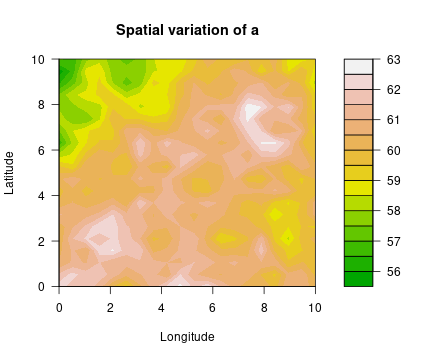filled.contour(unique(locs$x), unique(locs$y), matrix(exp(logb), ncol=sqrt(n_loc)),
color.palette = terrain.colors, xlab="Longitude", ylab="Latitude",
main="Spatial variation of b",
cex.lab=1,cex.axis=1)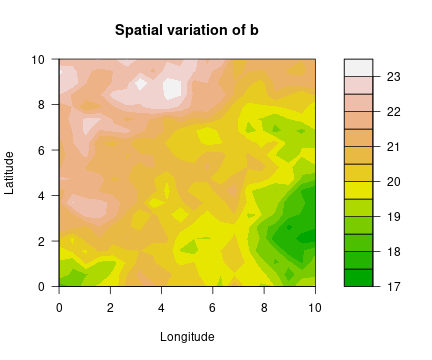filled.contour(unique(locs$x), unique(locs$y), matrix(exp(logs), ncol=sqrt(n_loc)),
color.palette = terrain.colors, xlab="Longitude", ylab="Latitude",
main="Spatial variation of s",
cex.lab=1,cex.axis=1)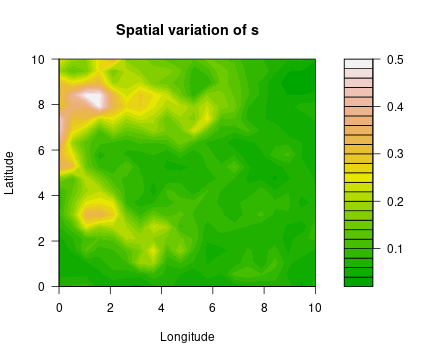Number of observations at each location is shown in the figure below.

barplot(sapply(y, length),
xlab = "Location", ylab = "Number of observations at each location",
main = "Summary of number of observations per location")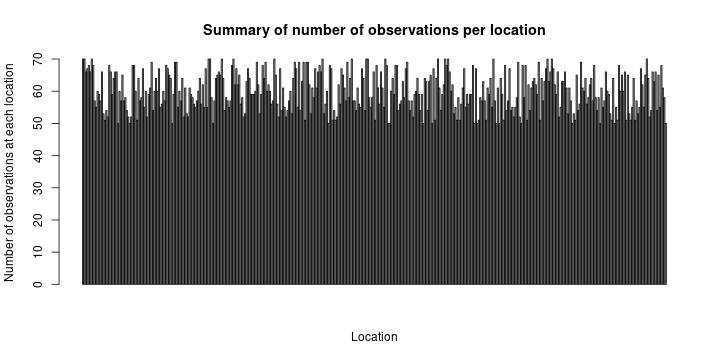Below are histograms of observations at $$8$$ randomly sampled locations.

set.seed(123)
n_sam <-8
sam_inds <- sample(1:n_loc, n_sam, replace=FALSE)
par(mfrow=c(2, n_sam/2))
for (i in sam_inds){
obs_i <- y[[i]]
hist(obs_i, breaks=8,
xlab="Observation value", main=paste("Observations at location", i))
}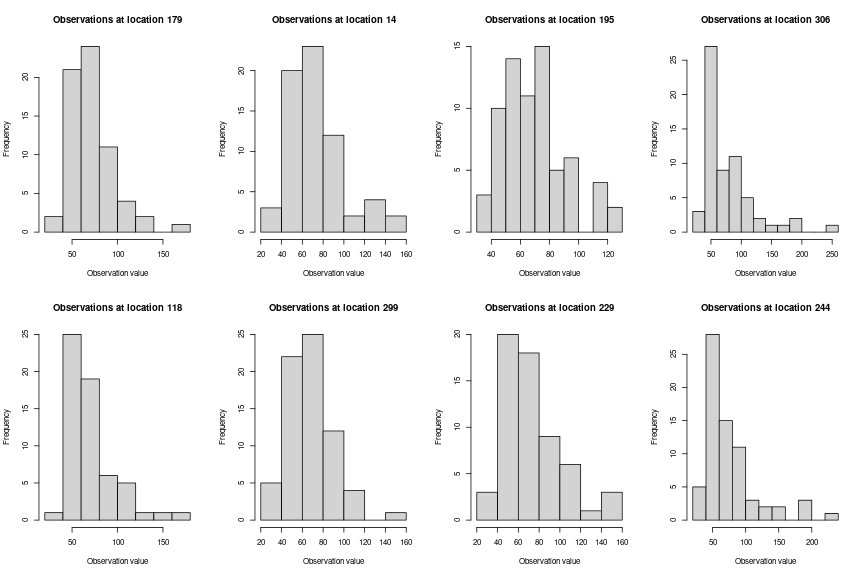### Model fitting

To fit the GEV-GP model to this simulated dataset, the first step is calling the spatialGEV_fit() function, for which several arguments must be provided:

• y: A list of $$n$$ vectors, each of which contains all data collected at one location.

• locs: A $$n \times 2$$ coordinate matrix.

• random: A character string indicating which GEV parameters are treated as spatial random effects. It can be one of “a”, “ab”, and “abs”, where “a”, “b”, and “s” represent the GEV location, scale, and shape parameters, respectively. Note that in the model $$b$$ is always estimated on the log scale since it is constrained to be positive.

• init_param: A list of initial parameters to be passed to the optimizer. Call ?spatialGEV_fit() and see Details for which parameters need to be included in the list.

• reparam_s: A flag for reparametrizing the GEV shape parameter $$s$$ - either “zero”, “unconstrained”, “negative”, or “positive”. For example, if reparam_s = "positive", the model works with the log-transformed shape parameter. Call ?spatialGEV_fit() and see Details for more information about this argument.

There are other arguments which user might want to specify to override the defaults:

• kernel: The kernel used for the Gaussian process(es) describing the spatial random effect(s). Currently 3 kernels are implemented: the default exponential kernel (kernel="exp"), the Matérn kernel (kernel="matern"), and the SPDE approximation to the Matérn kernel (kernel="spde") based on Lindgren, Rue, and Lindström (2011).

• X_a, X_b, X_s: Design matrices of covariates for the spatial random effects “a”, “b”, and “s”. The defaults are column matrices of $$1$$s, i.e., only an intercept parameter $$\beta_0$$ will be estimated for each spatial random effect.

• s_prior: A vector $$(\mu, \sigma)$$. Optionally a normal prior with parameters $$(\mu, \sigma)$$ can be specified on the fixed effect shape parameter $$s$$, or its reparametrized version depending on the value of reparam_s. When s_prior is not specified, a uniform prior is used.

• beta_prior: A named list of length-2 vectors $$(\mu, \sigma)$$. Optionally normal priors $$\mathcal{N}(\mu, \sigma^2)$$ can be put on the regression coefficients $$\boldsymbol{\beta}$$ for the random effects. To specify a normal prior on a random effect, name the vector beta_a or beta_b or beta_s. E.g., beta_prior=list(beta_a=c(0, 100)) puts a $$\mathcal{N}(0,100)$$ prior on $$a$$. When beta_prior is not specified, uniform priors are applied.

• matern_pc_prior: A named list with elements provided using the matern_pc_prior() function. Optionally penalized complexity priors can be put on the Matérn covariance hyperparameters. See ?matern_pc_prior() for more details.

The code below fits a GEV-GP model to the simulated data. The model assumes that all spatial random effects follow Gaussian processes with the exponential kernel: $k({\boldsymbol{x}}, {\boldsymbol{x}}') = \sigma^2\exp\left(-\frac{||{\boldsymbol{x}}-{\boldsymbol{x}}'||}{\ell}\right).$ No covariates are included in this model, so by default an intercept parameter $$\beta_0$$ is estimated for the GP of each spatial random effect.

fit <- spatialGEV_fit(y = y, locs = locs, random = "abs",
init_param = list(a = rep(60, n_loc),
log_b = rep(2,n_loc),
s = rep(-3,n_loc),
beta_a = 60, beta_b = 2, beta_s = -2,
log_sigma_a = 1.5, log_ell_a = 5,
log_sigma_b = 1.5, log_ell_b = 5,
log_sigma_s = -1, log_ell_s = 5),
reparam_s = "positive", kernel="exp", silent = T)
class(fit)
#>  "spatialGEVfit"
print(fit)
#> Model fitting took 161.277403354645 seconds
#> The model has reached relative convergence
#> The model uses a exp kernel
#> Number of fixed effects in the model is 9
#> Number of random effects in the model is 1200
#> Hessian matrix is positive definite. Use spatialGEV_sample to obtain posterior samples

### Posterior sampling

To obtain posterior samples of $$a$$, $$b$$, and $$s$$, we pass the fitted model object fit to spatialGEV_sample(), which takes in three arguments:

• model: An object of class spatialGEVfit, which is the output of spatialGEV_fit().

• n_draw: Number of samples to draw from the posterior distribution $$p(a, b, s \mid {\boldsymbol{Y}}, {\boldsymbol{X}})$$.

• observation: If set to TRUE, the function will also draw from the posterior predictive distribution $$p(y^{\mathrm{rep}} \mid {\boldsymbol{Y}})$$ at the observed locations. This is useful for Bayesian model checking, which we will demonstrate shortly.

• loc_ind: A vector of location indices at which samples will be drawn. Default is all locations.

The following line of code draws 2000 samples from the posterior distribution and the posterior predictive distribution:

sam <- spatialGEV_sample(model = fit, n_draw = 2000, observation = T)
print(sam)
#> The samples contains 2000 draws of 1209 parameters
#> The samples contains 2000 draws of response at 400 locations
#> Use summary() to obtain summary statistics of the samples

Then use summary() to view the summary statistics of the posterior samples.

pos_summary <- summary(sam)
pos_summary$param_summary[c(1:5, (n_loc+1):(n_loc+5), (2*n_loc+1):(2*n_loc+5), (3*n_loc):(nrow(pos_summary$param_summary))),]
#>                    2.5%        25%       50%       75%      97.5%       mean
#> a1          60.37275783 61.5980928 62.210164 62.835360 63.9804803 62.2141060
#> a2          60.75855130 61.7379378 62.328065 62.859586 63.9297423 62.3079065
#> a3          60.81983443 61.9089171 62.431818 62.940149 63.9610251 62.4305312
#> a4          60.79951615 61.8344749 62.345769 62.881036 63.8523161 62.3527635
#> a5          60.74117629 61.7295232 62.198760 62.745477 63.7537897 62.2326125
#> log_b1       2.93220334  2.9719966  2.991997  3.014360  3.0553563  2.9930003
#> log_b2       2.93448172  2.9723931  2.992898  3.011996  3.0494946  2.9924037
#> log_b3       2.94528959  2.9782006  2.996280  3.014380  3.0515383  2.9964359
#> log_b4       2.94725158  2.9830574  2.999908  3.017881  3.0510366  2.9998425
#> log_b5       2.95743662  2.9905772  3.008068  3.025838  3.0566551  3.0079155
#> s1          -3.86044147 -3.2227490 -2.921353 -2.609795 -2.0274468 -2.9200414
#> s2          -3.80428531 -3.2219404 -2.931187 -2.610808 -2.0574936 -2.9274155
#> s3          -3.76864653 -3.2296526 -2.950027 -2.664731 -2.1404922 -2.9526005
#> s4          -3.71662682 -3.1967825 -2.940971 -2.666217 -2.1067600 -2.9334609
#> s5          -3.67186841 -3.1723053 -2.923136 -2.676004 -2.2098599 -2.9288787
#> s400        -3.45638367 -2.8673479 -2.571656 -2.296527 -1.7403831 -2.5842184
#> beta_a      57.52397467 58.9584185 59.670918 60.446429 61.9572194 59.7032630
#> beta_b       2.90031130  2.9653742  3.001447  3.035666  3.1069824  3.0004916
#> beta_s      -3.19469003 -2.7156268 -2.459331 -2.212726 -1.7199864 -2.4620612
#> log_sigma_a -0.48482824  0.4901036  1.081240  1.610590  2.6947695  1.0606942
#> log_ell_a    0.00258028  1.3207450  2.001316  2.668811  3.9966672  1.9918314
#> log_sigma_b -7.24392340 -6.0568859 -5.344071 -4.671646 -3.4885019 -5.3640991
#> log_ell_b   -0.22138081  1.4165251  2.310469  3.212735  4.8795805  2.3244647
#> log_sigma_s -1.83110995 -1.0777626 -0.689746 -0.312738  0.3793501 -0.7062119
#> log_ell_s    0.08662430  0.9420228  1.363870  1.809115  2.6334788  1.3711216
pos_summary$y_summary[1:5,] #> 2.5% 25% 50% 75% 97.5% mean #> y1 37.54193 57.15973 70.41631 89.40103 147.7703 76.44984 #> y2 37.37216 55.66691 69.70116 86.28537 143.6716 74.30785 #> y3 38.43884 56.23423 70.12602 89.56860 151.4831 76.44669 #> y4 37.20683 56.01811 70.36766 89.65431 144.2591 75.52356 #> y5 36.58043 55.96459 70.51883 89.12971 147.1605 75.66020 ### Model checking Since we know the true values of $$a$$, $$b$$, and $$s$$ in this simulation study, we are able to compare the posterior mean with the true values. The posterior means of $$a$$, $$b$$ and $$s$$ at different locations are plotted against the true values below. par(mfrow=c(1,3)) plot(a, pos_summary$param_summary[1:n_loc,"mean"],
xlab="True a",
ylab="Posterior mean of a",
main="True vs Posterior Mean of a")
abline(0, 1, col="blue", lty="dashed")
plot(exp(logb), exp(pos_summary$param_summary[(n_loc+1):(2*n_loc),"mean"]), xlab="True b", ylab="Posterior mean of b", main="True vs Posterior Mean of b") abline(0, 1, col="blue", lty="dashed") plot(exp(logs), exp(pos_summary$param_summary[(2*n_loc+1):(3*n_loc),"mean"]),
xlab="True s",
ylab="Posterior mean of s",
main="True vs Posterior Mean of s")
abline(0, 1, col="blue", lty="dashed")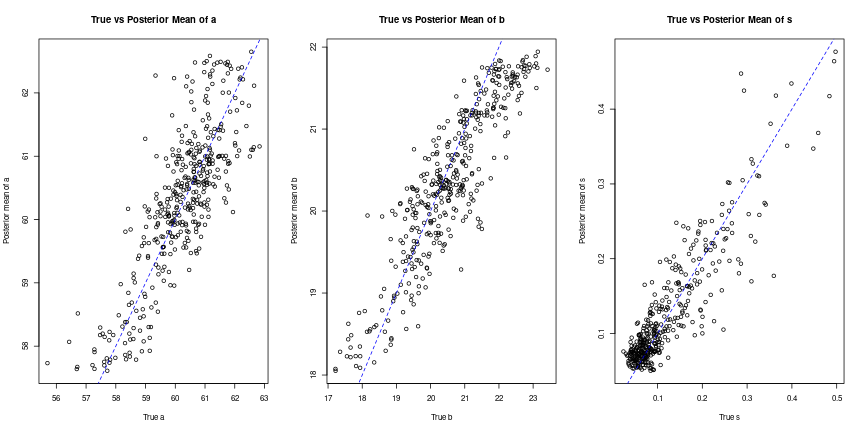### Posterior prediction

Finally, we demonstrate how to make predictions at new locations. This is done using the spatialGEV_predict() function, which takes the following arguments:

• model: An object of class spatialGEVfit, which is the output of spatialGEV_fit().

• locs_new: An $$m \times 2$$ matrix of the coordinates of the $$m$$ test locations.

• n_draw: Number of samples to draw from the posterior predictive distribution $$p({\boldsymbol{Y}}^{\mathrm{new}} \mid {\boldsymbol{Y}})$$.

• X_a_new, X_b_new, X_s_new: Design matrices for the spatial random effects. Need to be provided here if design matrices were used in model fitting.

• parameter_draws: An optional n_draws x n_parameters matrix that contains the posterior samples of all parameters. If spatialGEV_sample() has already been called prior to spatialGEV_predict(), its output can be provided to the prediction function to save time.

We randomly sample 100 locations from the simulated dataset simulatedData as test locations which are left out. Data from the rest 300 training locations are used for model fitting.

a <- simulatedData$a logb <- simulatedData$logb
logs <- simulatedData$logs y <- simulatedData$y
locs <- simulatedData$locs n_loc <- nrow(locs) In this dataset, the GEV parameters $$a$$ and $$\log(b)$$ are generated from the surfaces below, and the shape parameter is a constant $$\exp(-2)$$ across space. filled.contour(unique(locs$x), unique(locs$y), matrix(a, ncol=sqrt(n_loc)), color.palette = terrain.colors, xlab="Longitude", ylab="Latitude", main="Spatial variation of GEV location parameter a", cex.lab=1,cex.axis=1)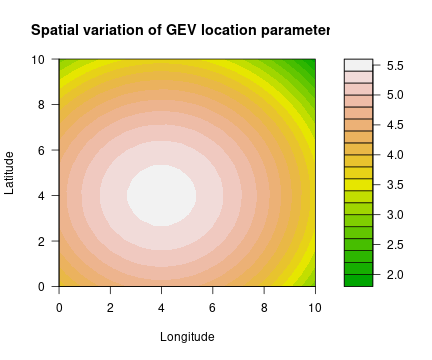filled.contour(unique(locs$x), unique(locs$y), matrix(exp(logb), ncol=sqrt(n_loc)), color.palette = terrain.colors, xlab="Longitude", ylab="Latitude", main="Spatial variation of GEV scale parameter b", cex.lab=1,cex.axis=1)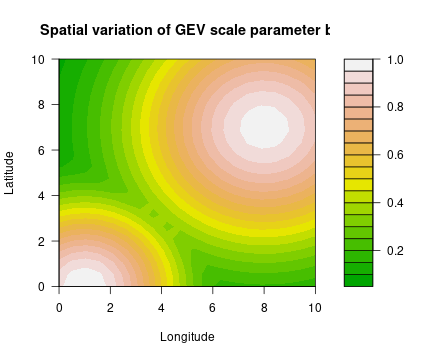We fit a GEV-GP model with random $$a$$ and $$b$$ and log-transformed $$s$$ to the training dataset. The kernel used here is the SPDE approximation to the Matérn covariance function (Lindgren, Rue, and Lindström (2011)). set.seed(123) n_test <- 100 n_train <- n_loc - n_test test_ind <- sample(1:n_loc, n_test) train_ind <- setdiff(1:n_loc, test_ind) # Obtain coordinate matrices and data lists locs_test <- locs[test_ind,] y_test <- y[test_ind] locs_train <- locs[-test_ind,] y_train <- y[-test_ind] # Fit the GEV-GP model to the training set train_fit_s <- spatialGEV_fit(y = y_train, locs = locs_train, random = "ab", init_param = list(a = rep(0, n_train), log_b = rep(0,n_train), s = 0, beta_a = 0, beta_b = 0, log_sigma_a = 1, log_kappa_a = -2, log_sigma_b = 1, log_kappa_b = -2), reparam_s = "positive", kernel="spde", silent = T) We see that using the SPDE approximation greatly speeds up model fitting: train_fit_s #> Model fitting took 12.7009427547455 seconds #> The model has reached relative convergence #> The model uses a spde kernel #> Number of fixed effects in the model is 7 #> Number of random effects in the model is 680 #> Hessian matrix is positive definite. Use spatialGEV_sample to obtain posterior samples The fitted model object is passed to spatialGEV_predict() for 2000 samples from the posterior predictive distributions. Note that this might take some time. pred_s <- spatialGEV_predict(model = train_fit_s, locs_new = locs_test, n_draw = 2000) pred_s #> 2000 posterior predictive samples have been draw for 100 test locations #> The number of training locations is 300 #> Use summary() to obtain summary statistics of the posterior predictive samples Then we call summary() on the pred object to obtain summary statistics of the posterior predictive samples at the test locations. pred_summary <- summary(pred_s) pred_summary[1:5,] #> 2.5% 25% 50% 75% 97.5% mean #> [1,] 3.176798 3.794829 4.249496 4.926165 7.320891 4.497156 #> [2,] 4.027011 4.227374 4.373467 4.553830 5.191482 4.428422 #> [3,] 3.976990 4.663539 5.221210 5.965266 8.307825 5.455898 #> [4,] 4.150172 4.505835 4.761149 5.101577 6.258151 4.873551 #> [5,] 3.541705 4.033024 4.423469 4.955809 6.754725 4.613262 Since we have the true observations at the test locations, we can compare summary statistics of the true observations to those of the posterior predictive distributions. In the figures below, each circle represents a test location. par(mfrow=c(1,2)) plot(sapply(y_test, mean), pred_summary[,"mean"], xlab="Test statistic from test data", ylab="Test statistic from predictive distribution", main="Test statistic = mean") abline(0, 1, col="blue", lty="dashed") plot(sapply(y_test, function(x){quantile(x, probs=0.975)}), pred_summary[,"97.5%"], xlab="Test statistic from test data", ylab="Test statistic from predictive distribution", main="Test statistic = 97.5% quantile") abline(0, 1, col="blue", lty="dashed")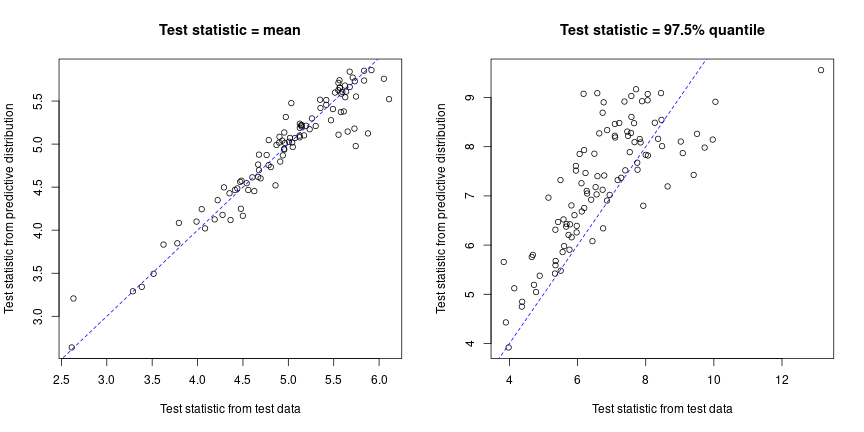## Case study: Yearly maximum snowfall data in Ontario, Canada In this section, we show how to use the SpatialGEV package to analyze a real dataset. The data used here are the 1987-2021 monthly total snowfall data (in cm) obtained from Environment and Natural Resources, Government of Canada. The link to download the raw data is https://climate-change.canada.ca/climate-data/#/monthly-climate-summaries. This dataset is automatically loaded with the package and is named ONsnow. library(dplyr) #library(maps) lon_range <- c(-96, -73) lat_range <- c(41.5, 55) summary(ONsnow) #> LATITUDE LONGITUDE STATION_NAME CLIMATE_IDENTIFIER LOCAL_YEAR LOCAL_MONTH TOTAL_SNOWFALL #> Min. :41.75 Min. :-94.62 Length:63945 Length:63945 Min. :1987 Min. : 1.000 Min. : 0.00 #> 1st Qu.:43.58 1st Qu.:-81.50 Class :character Class :character 1st Qu.:1991 1st Qu.: 3.000 1st Qu.: 0.00 #> Median :44.23 Median :-79.93 Mode :character Mode :character Median :1997 Median : 6.000 Median : 1.00 #> Mean :44.96 Mean :-80.88 Mean :1999 Mean : 6.469 Mean : 15.23 #> 3rd Qu.:45.50 3rd Qu.:-78.97 3rd Qu.:2005 3rd Qu.: 9.000 3rd Qu.: 23.60 #> Max. :54.98 Max. :-74.47 Max. :2021 Max. :12.000 Max. :326.00 maps::map(xlim = lon_range, ylim = lat_range) points(ONsnow$LONGITUDE, ONsnow$LATITUDE)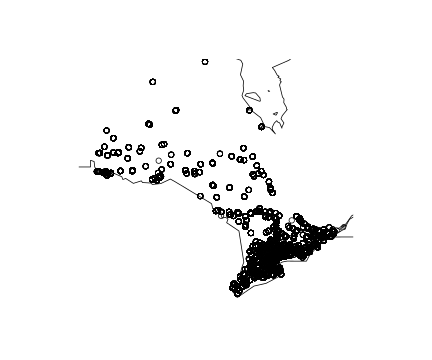### Data preprocessing We first grid the data using cells of length $$0.5^{\circ}$$. By doing this, weather stations that are apart by less than $$0.5^{\circ}$$ in longitude/latitude are grouped together in the same grid cell. From now on, we refer to each grid cell as a location. grid_locs <- grid_location(ONsnow$LONGITUDE, ONsnow$LATITUDE, sp.resolution = 0.5) data_grid <- cbind(grid_locs, ONsnow) data_grid[1:5,] #> cell_ind cell_lon cell_lat LATITUDE LONGITUDE STATION_NAME CLIMATE_IDENTIFIER LOCAL_YEAR LOCAL_MONTH TOTAL_SNOWFALL #> 1 259 -89.867 54 53.833 -89.867 BIG TROUT LAKE 6010738 1987 1 33.6 #> 2 259 -89.867 54 53.833 -89.867 BIG TROUT LAKE 6010738 1987 2 33.6 #> 3 259 -89.867 54 53.833 -89.867 BIG TROUT LAKE 6010738 1987 3 19.8 #> 4 259 -89.867 54 53.833 -89.867 BIG TROUT LAKE 6010738 1987 4 5.6 #> 5 259 -89.867 54 53.833 -89.867 BIG TROUT LAKE 6010738 1987 5 12.8 For each location, we find the maximum snowfall amount each year and only keep locations where there are at least two years of records. # Yearly max for each location all_locs <- data_grid %>% select(cell_ind, cell_lon, cell_lat) %>% distinct() yearly_max_records <- data_grid %>% group_by(cell_ind, LOCAL_YEAR) %>% slice(YEARLY_MAX_SNOWFALL = which.max(TOTAL_SNOWFALL)) %>% select(cell_ind, LOCAL_YEAR, LOCAL_MONTH, TOTAL_SNOWFALL) %>% rename(YEARLY_MAX_SNOWFALL = TOTAL_SNOWFALL) %>% filter(YEARLY_MAX_SNOWFALL > 0) %>% # Remove records of 0s left_join(all_locs, by="cell_ind") # Coordinates of the locations locs <- yearly_max_records %>% ungroup() %>% select(cell_ind, cell_lon, cell_lat) %>% distinct() n_loc <- nrow(locs) # Make data into a list in which each vector contains data from one location Y <- vector(mode="list", length=n_loc) for (i in 1:n_loc){ id <- locs$cell_ind[i]
Y[[i]] <- yearly_max_records %>%
ungroup() %>%
filter(cell_ind==id) %>%
pull(YEARLY_MAX_SNOWFALL)
}

# Only keep locations with at least 2 years of records
chosen_loc_ind <- which(sapply(Y, length) >= 2)
Y <- Y[chosen_loc_ind]
locs <- locs %>% select(cell_lon, cell_lat) %>% slice(chosen_loc_ind)
n_loc <- nrow(locs)
maps::map(xlim = lon_range, ylim = lat_range)
points(locs$cell_lon, locs$cell_lat)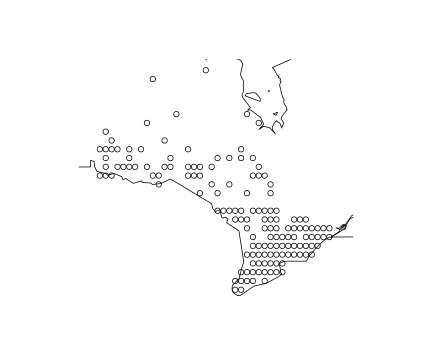Now we fit the GEV-GP model to the data using the exponential kernel function. Both $$a$$ and $$b$$ are treated as spatial random effects. $$s$$ is constrained to be a positive constant. Note that here we have specified a $$\mathcal{N}(-5,5)$$ prior on the log-transformed shape parameter. This is because we found that the shape parameter is estimated close to 0 and such a prior ensures model fitting procedure is numerically stable.

fit <- spatialGEV_fit(Y, locs, random="ab",
init_param = list(a=rep(55, n_loc),
log_b=rep(3, n_loc),
s=-5,
beta_a=55, beta_b=3,
log_sigma_a=6, log_ell_a=-1,
log_sigma_b=0, log_ell_b=-1),
reparam_s="positive",
kernel="exp",
s_prior=c(-5,5),
silent=T)
fit
#> Model fitting took 6.4646999835968 seconds
#> The model has reached relative convergence
#> The model uses a exp kernel
#> Number of fixed effects in the model is 7
#> Number of random effects in the model is 286
#> Hessian matrix is positive definite. Use spatialGEV_sample to obtain posterior samples

Next, 5000 samples are drawn from the joint posterior distribution of all parameters.

sam <- spatialGEV_sample(fit, n_draw=5000, observation=F)

From the samples we can calculate the 5-year, 10-year, and 25-year return levels at all locations, which are the upper $$1/5$$%, $$1/10$$%, and $$1/25$$% quantiles of the extreme value distributions at these locations.

#library(evd)
p_draws <- sam$parameter_draws # Get indices of each GEV parameter in the sample matrix a_ind <- which(colnames(p_draws)%in% paste0("a", 1:n_loc)) logb_ind <- which(colnames(p_draws)%in% paste0("log_b", 1:n_loc)) logs_ind <- which(colnames(p_draws) == "s") # Deine a function to draw from the return level posterior distribution rl_draw <- function(return_period){ apply(p_draws, 1, function(all_draw){ mapply(evd::qgev, p=1/return_period, loc=all_draw[a_ind], scale=exp(all_draw[logb_ind]), shape=exp(all_draw[logs_ind]), lower.tail=F) }) } # 5 year return-level q5_draws <- rl_draw(5) return5 <- apply(q5_draws, 1, mean) return5sd <- apply(q5_draws, 1, sd) # 10 year return-level q10_draws <- rl_draw(10) return10 <- apply(q10_draws, 1, mean) return10sd <- apply(q10_draws, 1, sd) # 25 year return-level q25_draws <- rl_draw(25) return25 <- apply(q25_draws, 1, mean) return25sd <- apply(q25_draws, 1, sd) Plotted below are the return levels. map_plot <- function(value, zlim=NULL){ if (is.null(zlim)) zlim=range(value) maps::map("world", xlim=lon_range, ylim = lat_range, col="white", fill=TRUE) fields::image.plot(legend.only = T, zlim=zlim, col=viridisLite::viridis(10), horizontal = TRUE, legend.shrink = 0.5, cex=0.7, smallplot=c(0.4, 0.9, 0.1, 0.14)) val = fields::color.scale(value, col=scales::alpha(viridisLite::viridis(10), 1), zlim=zlim) points(locs$cell_lon, locs\$cell_lat, col=val, pch=15, cex=0.9)
}
map_plot(return5)
title("5-year Return levels")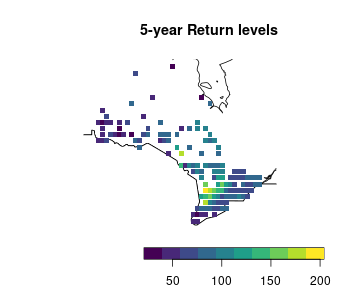map_plot(return5sd)
title("SD of 5-year Return levels")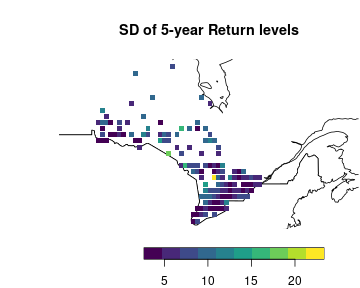map_plot(return10)
title("10-year Return levels")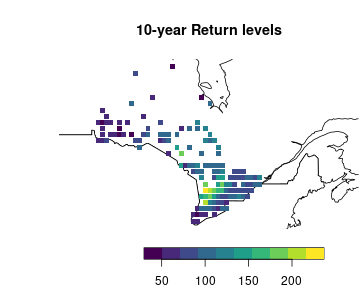map_plot(return10sd)
title("SD of 10-year Return levels")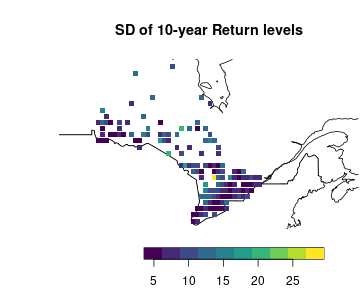map_plot(return25)
title("25-year Return levels")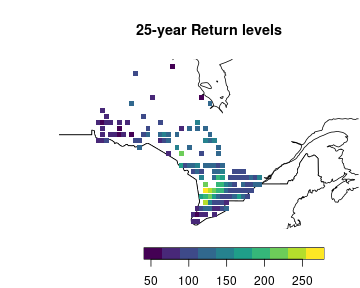map_plot(return25sd)
title("SD of 25-year Return levels")